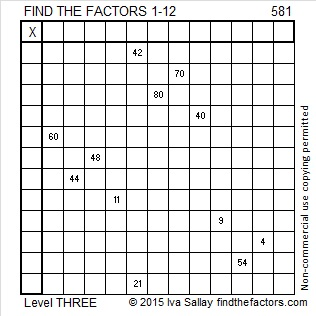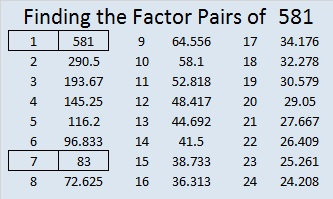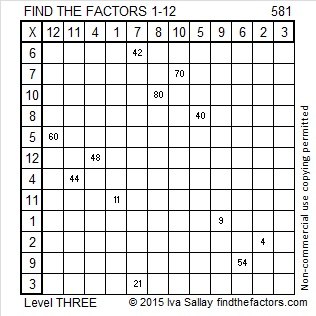# 581 and Level 3

A year ago I wrote a short, but heartfelt post for World Elephant Day. I invite you to read it or any other article that makes you aware of what you can do to protect elephants from poachers.

581 is the sum of consecutive primes two different ways: 191 + 193 + 197 = 581, and the sum of all the prime numbers from 19 to 71 also is 581.Print the puzzles or type the solution on this excel file: 12 Factors 2015-08-10

—————————————————————————————————

• 581 is a composite number.
• Prime factorization: 581 = 7 x 83
• The exponents in the prime factorization are 1 and 1. Adding one to each and multiplying we get (1 + 1)(1 + 1) = 2 x 2 = 4. Therefore 581 has exactly 4 factors.
• Factors of 581: 1, 7, 83, 581
• Factor pairs: 581 = 1 x 581 or 7 x 83
• 581 has no square factors that allow its square root to be simplified. √581 ≈ 24.10394—————————————————————————————————

A Logical Approach to solve a FIND THE FACTORS puzzle: Find the column or row with two clues and find their common factor. Write the corresponding factors in the factor column (1st column) and factor row (top row).  Because this is a level three puzzle, you have now written a factor at the top of the factor column. Continue to work from the top of the factor column to the bottom, finding factors and filling in the factor column and the factor row one cell at a time as you go.This site uses Akismet to reduce spam. Learn how your comment data is processed.